# Pi facts for kids

Kids Encyclopedia Facts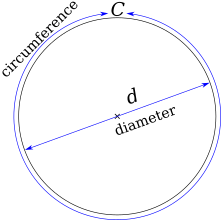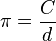$\pi = \frac {C}{d}$ (pi is equal to the circumference divided by the diameter).

Pi, or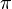$\pi$, is a mathematical constant. It is the ratio of the distance around a circle to the circle's diameter. This produces a number, and that number is always the same. However, the number is rather strange. The number starts 3.14159265... and continues without end. Numbers like this are called irrational numbers.

The diameter is the longest line which can be fitted inside a circle. It passes through the center of the circle. The distance around a circle is known as the circumference. Even though the diameter and circumference are different for different circles, the number pi remains constant: its value never changes. This is because the relationship between the circumference and diameter is always the same.

## Approximation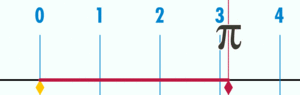A diagram showing how$\pi$ can be found by using a circle with a diameter of one. The circumference of this circle is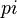$pi$.

Pi is often written formally as$\pi$ or the Greek letter π as a shortcut. Pi is also an irrational number, meaning it cannot be written as a fraction (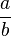$a \over b$), where 'a' and 'b' are integers (whole numbers). This basically means that the digits of pi that are to the right of the decimal go forever without repeating in a pattern, and that it is impossible to write the exact value of pi as a number. Pi can only be approximated, or measured to a value that is close enough for practical purposes.

A value close to pi is 3.1415926535897932384626433... A common fraction approximation of pi is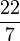$22 \over 7$, which yields approximately 3.14285714. This approximation is 0.04% away from the true value of pi. While this approximation is accepted for most of its use in real life, the fraction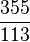$355 \over 113$ is more accurate (giving about 3.14159292), and can be used when a value closer to pi is needed. Computers can be used to get better approximations of pi.

== Historical The value of pi was known to Ancient Indian mathematicians like Bhaskaracharya and Aryabhatt.

Mathematicians have known about pi for thousands of years because they have been working with circles for the same amount of time. Civilizations as old as the Babylonians have been able to approximate pi to many digits, such as the fraction 25/8 and 256/81. Most historians believe that ancient Egyptians had no concept of π and that the correspondence is a coincidence.

The first written reference to pi dates to 1900 BC. Around 1650 BC the Egyptian Ahmes gave a value in the Rhind Papyrus. The Babylonians were able to find that the value of pi was slightly greater than 3 by simply making a big circle and then sticking a piece of rope onto the circumference and the diameter, taking note of their distances, and then dividing the circumference by the diameter.

Knowledge of the number pi passed back into Europe and into the hands of the Hebrews, who made the number important in a section of the Bible called the Old Testament. After this, the most common way of trying to find pi was to draw a shape of many sides inside any circle and use the area of the shape to find pi. The Greek philosopher Archimedes, for example, used a polygon shape that had 96 sides in order to find the value of pi, but the Chinese in 500 A.D. were able to use a polygon with 16,384 sides to find the value of pi. The Greeks, like Anaxagoras of Clazomenae, were also busy with finding out other properties of the circle, such as how to make squares of circles and squaring the number pi. Since then, many people have been trying to find out more and more exact values of pi.

A history of pi
Philosopher Date Approximation
Ptolemy around 150 A.D. 3.1416
al-Khwarizmi around 800 A.D. 3.1416
al-Kashi around 1430 A.D. 3.14159265358979
Viète 1540–1603 3.141592654
Roomen 1561–1615 3.14159265358979323
Van Ceulen around 1600 A.D. 3.14159265358979323846264338327950288

In the 16th century, better and better ways of finding pi became available such as the complicated formula that the French lawyer François Viète developed. The first use of the Greek symbol "π" was in an essay written in 1706 by William Jones.

A mathematician named Lambert also showed in 1761 that the number pi was irrational; that is, it cannot be written as a fraction by normal standards. Another mathematician named Lindeman was also able to show in 1882 that pi was part of the group of numbers known as transcendentals, which are numbers that cannot be the solution to a polynomial equation.

Pi can also be used for figuring out many other things beside circles. The properties of pi have allowed it to be used in many other areas of math besides geometry, which studies shapes. Some of these areas are complex analysis, trigonometry, and series.

## Pi in real life

Today, there are different ways to calculate many digits of$\pi$. This is of limited use though.

Pi can sometimes be used to work out the area or the circumference of any circle. To find the circumference of a circle, use the formula C (circumference) = π times diameter. To find the area of a circle, use the formula π (radius²). This formula is sometimes written as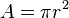$A = \pi r^2$, where r is the variable for the radius of any circle and A is the variable for the area of that circle.

To calculate the circumference of a circle with an error of 1 mm:

• 4 digits are needed for a radius of 30 meters
• 10 digits for a radius equal to that of the earth
• 15 digits for a radius equal to the distance from the earth to the sun.

People generally celebrate March 14 as Pi Day because March 14 is also written as 3/14, which represents the first three numbers 3.14 in the approximation of pi.

## Images for kidsPi Facts for Kids. Kiddle Encyclopedia.